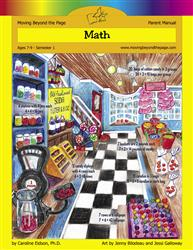# Common Core Alignment

CCSS.Math.Content.3.OA.1 - Interpret products of whole numbers, e.g., interpret 5 × 7 as the total number of objects in 5 groups of 7 objects each. For example, describe a context in which a total number of objects can be expressed as 5 × 7.

## 5: MathUnit 1: Multiplication and Division I
Lesson 10: More Practice With 2, 3, 4, and 5
Lesson 11: Multiples of 10
Lesson 12: Problem Solving With Multiplication
Lesson 15: Unit Test
Lesson 2: What Is Multiplication?
Lesson 3: Arrays and Equal Groups
Lesson 4: Repeated Addition and Number Lines
Lesson 6: The Commutative Property of Multiplication
Lesson 7: Multiples of 2 and 3
Lesson 8: More Practice With 2 and 3
Lesson 9: Multiples of 4 and 5
Final Project: Multiples Mini-Posters